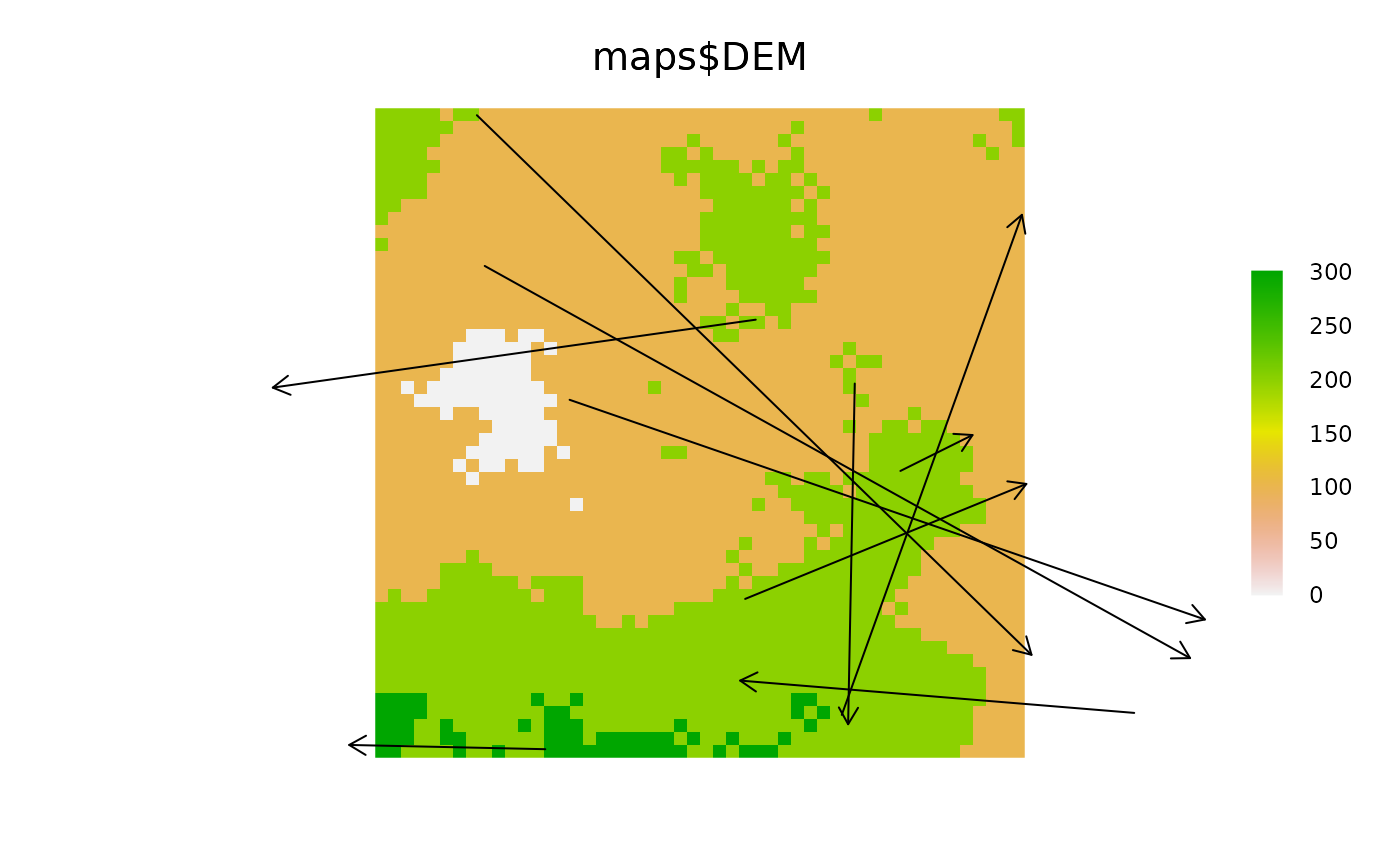The primary conceived usage of this is to draw arrows following the trajectories of agents.

makeLines(from, to)

# S4 method for SpatialPoints,SpatialPoints
makeLines(from, to)

## Arguments

from Starting spatial coordinates (SpatialPointsDataFrame). Ending spatial coordinates (SpatialPointsDataFrame).

## Value

A SpatialLines object. When this object is used within a Plot call and the length argument is specified, then arrow heads will be drawn. See examples.

## Examples

library(sp)
library(raster)

# Make 2 objects
caribou1 <- SpatialPoints(cbind(x = stats::runif(10, -50, 50),
y = stats::runif(10, -50, 50)))
caribou2 <- SpatialPoints(cbind(x = stats::runif(10, -50, 50),
y = stats::runif(10, -50, 50)))

caribouTraj <- makeLines(caribou1, caribou2)

clearPlot()Plot(caribouTraj, length = 0.1)# or  to a previous Plot
files <- dir(system.file("maps", package = "quickPlot"), full.names = TRUE, pattern = "tif")
maps <- lapply(files, raster)
names(maps) <- lapply(maps, names)

caribouTraj <- makeLines(caribou1, caribou2)

clearPlot()Plot(maps$DEM)Plot(caribouTraj, addTo = "maps$DEM", length = 0.1)clearPlot()